# Difference cochain and chain

(diff) ← Older revision | Latest revision (diff) | Newer revision → (diff)

A difference cochain is an obstruction to the extension of a homotopy between mappings. Letbe some cellular space, letbe a simply-connected topological space and suppose, moreover, that one is given two mappingsand a homotopy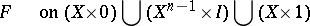(where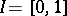and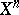is the-dimensional skeleton of) between these mappings on the-dimensional skeleton. For every oriented-dimensional cellof, the restriction oftogives a mapping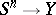(is the-dimensional sphere) and hence an element of the group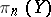. Thus there arises the cochain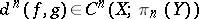(the notation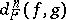would be more precise), which is called the difference cochain; the cochain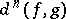is an obstruction to the extension ofto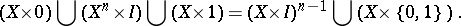The following statements hold: 1)if and only if the homotopy betweenandcan be extended to; 2) the cochainis a cocycle; 3) the cohomology class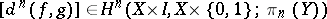vanishes if and only if there is a homotopy betweenandonthat coincides withon. Without loss of generality one can assume thatandcoincide on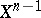and that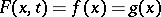for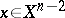. Then the following statements hold:

1), in particular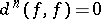;

2)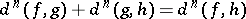;

3) for any mappingand for any cochain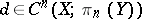there is a mappingfor which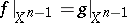and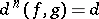.

Now suppose one is given two mappings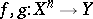,and let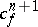and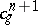be the obstructions to the extensions of the corresponding mappings. The role of the difference cochain in the theory of obstructions is explained by the following proposition:Thus, ifcan be extended to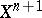, then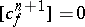and if, then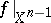can be extended to.